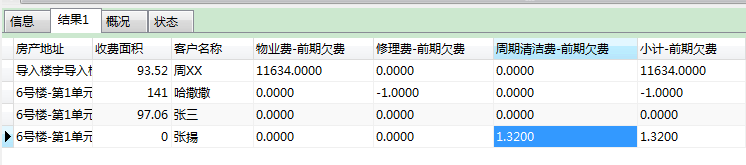sql中变量可以定义别名么

foreach collection="itemIdNames" item="item" index="index" open="" close="" separator=""
sum(if(b.item_id =SUBSTRING_INDEX(#{item,jdbcType=VARCHAR} , '-', 1)

``````and  pay_tag = 0  and b.start_date <![CDATA[<]]>
date_format(CONCAT(STR_TO_DATE(#{currentDate,jdbcType=VARCHAR},'%Y-%m-%d'), ' 00:00:00'),'%Y-%m-%d %H:%i:%s'),b.fee , 0  ))
SUBSTRING_INDEX(#{item,jdbcType=VARCHAR},'-', -1),
</foreach>
sum(if(pay_tag = 0  and b.start_date <![CDATA[<]]>
date_format(CONCAT(STR_TO_DATE(#{currentDate,jdbcType=VARCHAR},'%Y-%m-%d'), ' 00:00:00'),'%Y-%m-%d %H:%i:%s'),b.fee , 0 ))
sumOweBefore,

itemIdNames是有 id-name构成，我希望sql查询出来对应一个别名。所以这里别名不能写死（类似name之类的，这样会报重复的错误）。

求大神指导该怎么办。
foreach加上<>后就无法显示  代码没有问题
``````1个回答